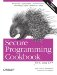# Converting Numbers to Strings

Problem

You have numeric types (int, float) and you need to put the results in a string, perhaps formatted a certain way.

Solution

There are a number of different ways to do this, all with benefits and drawbacks. The first technique I will present uses a stringstream class to store the string data, because it is part of the standard library and easy to use. This approach is presented in Example 3-3. See the discussion for alternative techniques.

Example 3-3. Formatting a number as a string

```#include
#include
#include
#include

using namespace std;

int main( ) {

stringstream ss;

ss << "There are " << 9 << " apples in my cart.";
cout << ss.str( ) << endl; // stringstream::str( ) returns a string
// with the contents

ss.str(""); // Empty the string
ss << showbase << hex << 16; // Show the base in hexadecimal
cout << "ss = " << ss.str( ) << endl;

ss.str("");
ss << 3.14;
cout << "ss = " << ss.str( ) << endl;
}```

The output of Example 3-3 looks like this:

```There are 9 apples in my cart.
ss = 0x10
ss = 3.14```

Discussion

A stringstream is a convenient way to put data into a string because it lets you use all of the formatting facilities provided by the standard input and output stream classes. In the simplest case in Example 3-3, I just use the left-shift operator (<<) to write a combination of text and numeric data to my string stream:

`ss << "There are " << 9 << " apples in my cart.";`

The << operator is overloaded for built-in types to format the input accordingly. When you want to get the string that holds your data, use the str member function:

`cout << ss.str( ) << endl;`

There are lots of stream manipulators in , and you can use them to do all sorts of formatting of your numeric data as you put it in the string. I used showbase and hex to format my number as hexadecimal in Example 3-3, but there are lots more. For example, you can set the precision to display more than the default number of digits:

`ss << setprecision(6) << 3.14285;`

Using manipulators isn't the most intuitive thing though, which is why there is a Recipe on the subject. See Recipe Recipe 10.2 for more detailed information about formatting numeric data with stream manipulators.

Of course, as is often the case with C++, there is another way. The Boost Format library (written by Samuel Krempp) contains a format class that makes formatting and conversion extremely easy. Example 3-4 shows you how to do such a conversion.

Example 3-4. Formatting integers as hexadecimal

```#include
#include

using namespace std;
using boost::format;
using boost::io::str;
using boost::io::format_error;

int main( ) {

try {
format f("There are %1% ways %2% %3% %4%");

f % 3;
f % "to" % "do" % "this.";

cout << f << endl;

f.clear( ); // Clear buffers to format something else

f.parse("Those cost \$%d.");
f % 50;

cout << f << endl;

int x = 11256099;

string strx = str(format("%x") % x);
cout << strx << endl;
}
catch (format_error &e) {
cout << e.what( ) << endl;
}
}```

Here's what you see when you run this program:

```There are 3 ways to do this.
Those cost \$50.
abc123```

Using a format class involves two steps, creating the format object and then sending it the content. To use the trivial case from Example 3-4, I create the format object using the simplest version of its syntax:

`format f("There are %1% ways %2% %3% %4%");`

In the format string, the placeholders are numbers with a % on either side. Then I start sending it the content for the format I provided:

```f % 3;
f % "to" % "do" % "this.";```

The % operator has been overridden in the formatting library to add the variables you give it to the format object to its left. You can use it once per line or call it several times in a row. It is analogous to the << operator for streams. Speaking of the << operator, it has also been overridden so you can write format objects directly to an output stream. Alternatively, if you need to put the results in a string, use the str member function:

`string s = f.str( );`

If you are a printf person, you can use printf format specifiers:

```f.parse("Those cost \$%d.");
f % 50;```

If you feed too many or too few content variables to format and try to write it to a stream or extract a formatted string, it will throw a format_error (or a subclass thereof) exception.

The format class is quite powerful, and it has too many neat formatting capabilities to list here, but it's worth checking out. See Boost's web site at www.boost.org to download Boost or to read the documentation.

You can also convert numbers from numeric types to strings using sprintf or the related functions. Typically, you should avoid this because it is unsafe and there are better alternatives.

Chapter 10Secure Programming Cookbook for C and C++: Recipes for Cryptography, Authentication, Input Validation & More
ISBN: 0596003943
EAN: 2147483647
Year: 2006
Pages: 241